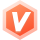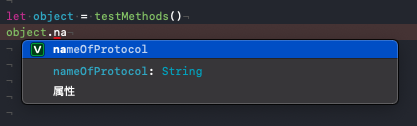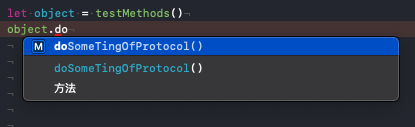# 理解Swift中的 some 不透明返回类型ReyZhang移动开发领域新星创作者
2023-05-31 10:07:57

## 一、概述

### 1.1 不透明类型 Opaque Return Types

`不透明类型`是指我们被告知对象的`功能`而不知道对象具体是什么`类型`

1. 具有不透明返回类型的`函数``方法`会隐藏返回值的类型信息。函数不再提供具体的类型作为返回类型，而是根据它支持的`协议`来描述返回值。
2. 在处理模块和调用代码之间的关系时，隐藏类型信息非常有用，因为返回的底层数据类型仍然可以保持私有。而且不同于返回`协议`类型，不透明类型能`保证类型一致性` —— `编译器`能获取到类型信息，同时`模块使用者`却不能获取到。

### 1.2 协议类型作为返回值

`协议类型`作为返回值时，返回对象仅能调用协议中定义的`属性``方法`。与返回值类型是否是不透明返回值类型没有关系

``````protocol MyProtocol {
///属性
var  nameOfProtocol:String {get set}
///方法
func doSomeTingOfProtocol () -> Void
}

struct MyStruct:MyProtocol {
///协议相关
var nameOfProtocol: String = "协议属性"
func doSomeTingOfProtocol() {
print("学习")
}

///自定义相关
var nameOfSelf:String = "自定义属性"
func doSomeTingOfSelf() {
print("打游戏")
}
}

func testMethods() -> some MyProtocol { ///返回遵守MyProtocol协议的类型
return MyStruct()
}
let object = testMethods()
``````### 1.3 语法

``````func makeA() -> some Equatable { ///返回类型是 some + Equatable协议 的不透明类型
"A"  ///单表达式函数的隐式返回，此处隐藏了return
}
``````

``````let a = makeA()
let anotherA = makeA()

print(a == anotherA) // ✅ The compiler knows that both values are strings
``````

``````func makeOne() -> some Equatable { ///返回类型是 some + Equatable协议 的不透明类型
1        ///单表达式函数的隐式返回，此处隐藏了return
}
let one = makeOne()
print(a == one) // ❌ Compilation error: `a` and `one` are of different types, although both conform to `Equatable`
``````

``````var x: Int = 0
x = makeOne() // ❌ Compilation error: Cannot assign value of type 'some Equatable' to type 'Int'
``````

``````func makeOneOrA(_ isOne: Bool) -> some Equatable {
isOne ? 1 : "A" // ❌ Compilation error: Cannot convert return expression of type 'Int' to return type 'some Equatable'
}
``````

## 二、不透明类型和泛型

`不透明类型`是一种`特殊的泛型`

`泛型`是用于`类型级别抽象`的Swift语言特性。 它允许将一种类型与满足给定`约束集`的任何其他类型`以相同的方式`使用。

### 2.1 泛型受`调用者`约束

``````func foo<T: Equatable>() -> T { ... }

let x: Int = foo() 　　 // T == Int, chosen by caller
let y: String = foo()  // T == String, chosen by caller
``````

### 2.2 不透明类型受`被调用者`约束

``````func bar() -> some Equatable { ... }
let z = bar() // z is abstracted to Equatable. Concrete type is chosen by bar() implementation
``````

## 三、不透明类型和协议

### 3.1 不能从函数返回带有`Self`或`associatedtype`要求的协议。 相反，不透明类型却可以：

``````// Equatable protocol declaration from the Swift standard library
public protocol Equatable {
static func == (lhs: Self, rhs: Self) -> Bool
}

func makeTwo() -> Equatable { 2 } // ❌ Protocol 'Equatable' can only be used as a generic constraint because it has Self or associated type requirements
func makeThree() -> some Equatable { 3 } // ✅
``````

`SwiftUI`中你会发现大量使用`some``associatedtype`修饰的语法。所以了解一下还是很有必要的。

### 3.2 一个函数可以返回`不同的协议类型`。 相反，它每次必须返回`相同的不透明类型`：

``````///自定义协议
protocol P {}
///拓展基础类型
extension Int: P {}
extension String: P {}
///区别
func makeIntOrString(_ isInt: Bool) -> P { isInt ? 1 : "1" } // ✅
func makeIntOrStringOpaque(_ isInt: Bool) -> some P { isInt ? 1 : "1" } // ❌ Compilation error
``````

## 四、不透明类型解决的问题

``````///协议：Shape
protocol Shape {}

/// Rectangle
struct Rectangle: Shape {}
/// Union
struct Union<A: Shape, B: Shape>: Shape {
var a: Shape
var b: Shape
}

/// Transformed
struct Transformed<S: Shape>: Shape {
var shape: S
}

///协议：GameObject
protocol GameObject {
associatedtype ShapeType: Shape
var shape: ShapeType { get }
}
/// EightPointedStar
struct EightPointedStar: GameObject {
var shape: Union<Rectangle, Transformed<Rectangle>> {
return Union(a:Rectangle(), b:Transformed(shape: Rectangle()))
}
}
``````

1. 上述代码是可以编译通过的，但是 EightPointedStar 的 shape 返回类型又臭又长，被暴露了出去；如果换成 Shape 则编译不通过，原因是 `associatedtype` ShapeType 要求必须指定`具体的类型`，而 Shape 不实现 Shape 本身。
2. 假如 Shape 协议中含有 `Self` 或者 `associatedtype`，无法作为函数的返回参数。这是 Swift 泛型系统长久以来的一个问题。

1. 语法上`隐藏具体类型`，所以叫做不透明结果类型
2. 强类型：类型参数不丢失
3. 允许带有 `Self` 或者 `associatedtype``protocol`作为`返回类型`

``````struct EightPointedStar: GameObject {
var shape: some Shape {
return Union(a:Rectangle(), b:Transformed(shape: Rectangle()))
}
}
``````

``````func foo<T: Equatable>(x: T, y: T) -> some Equatable {
let condition = x == y
return condition ? 42 : 11
}

let x = foo(x: "apples", y: "bananas")
let y = foo(x: "apples", y: "oranges")

print(x == y) // 这里可以被调用是因为泛型系统保留了强类型
``````

## 六、总结

1. 不透明类型可以被认为是具有私有`基础类型`的协议。
2. 不透明类型是由`函数实现者`定义的，而不是由`调用者定义`的。
3. 一个函数每次必须返回`相同的`不透明类型。
4. 允许带有`Self`或者 `associatedtype``protocol` 作为`返回类型`
...全文

527 回复 打赏 收藏 转发到动态 举报了解Swift的不透明返回类型Swift ui @state原理 some不透明类型
@state @state原理 访问权限 some不透明类型 协议只是一种宽泛的约束，虽然some类型也能返回不同类型，而在这里只返回特定的类型，不能根据不同的分支返回 ...Swift】不透明类型Swift的some关键字SwiftUI 和 Swift 5.1 新特性(4) 苹果先斩后奏？Function Builder 造就 SwiftUI 的 DSLSwift 泛型 Class 结构体、枚举 关联类型associatedtype 约束 不透明类型Some 约束复合条件where

423发帖与我相关我的任务
iosflutterandroid 技术论坛（原bbs） 山东省·青岛市• 近7日
• 近30日
• 至今